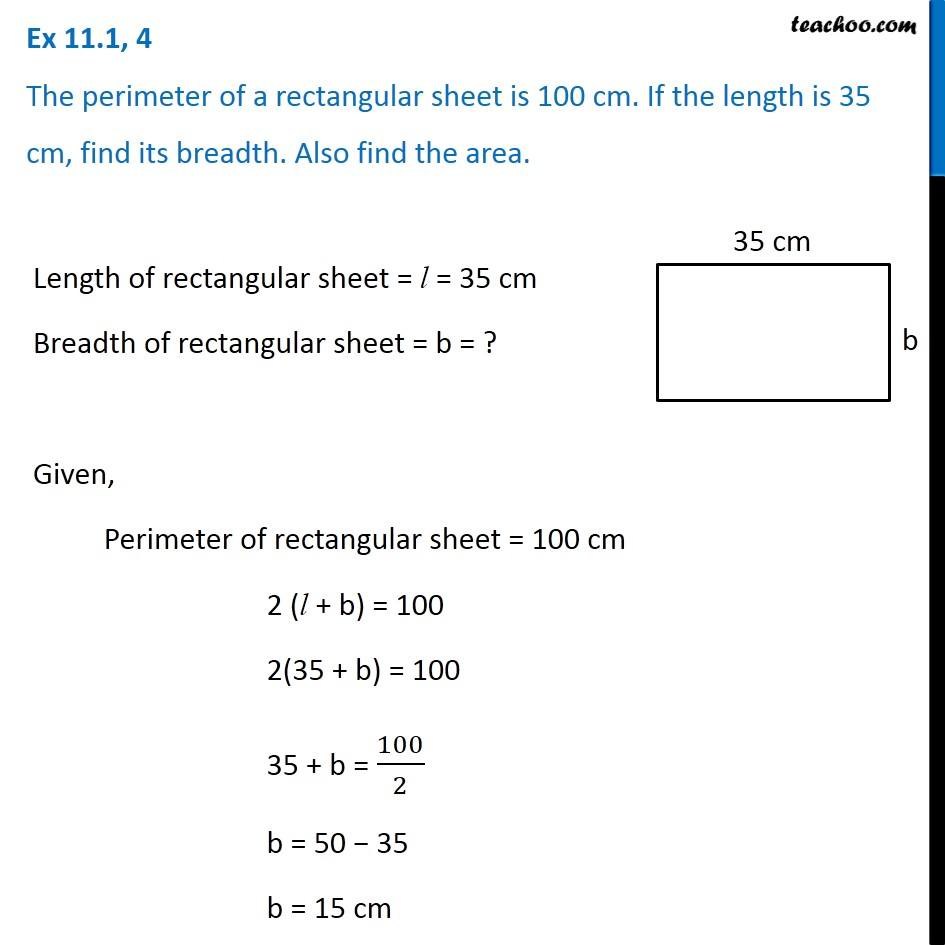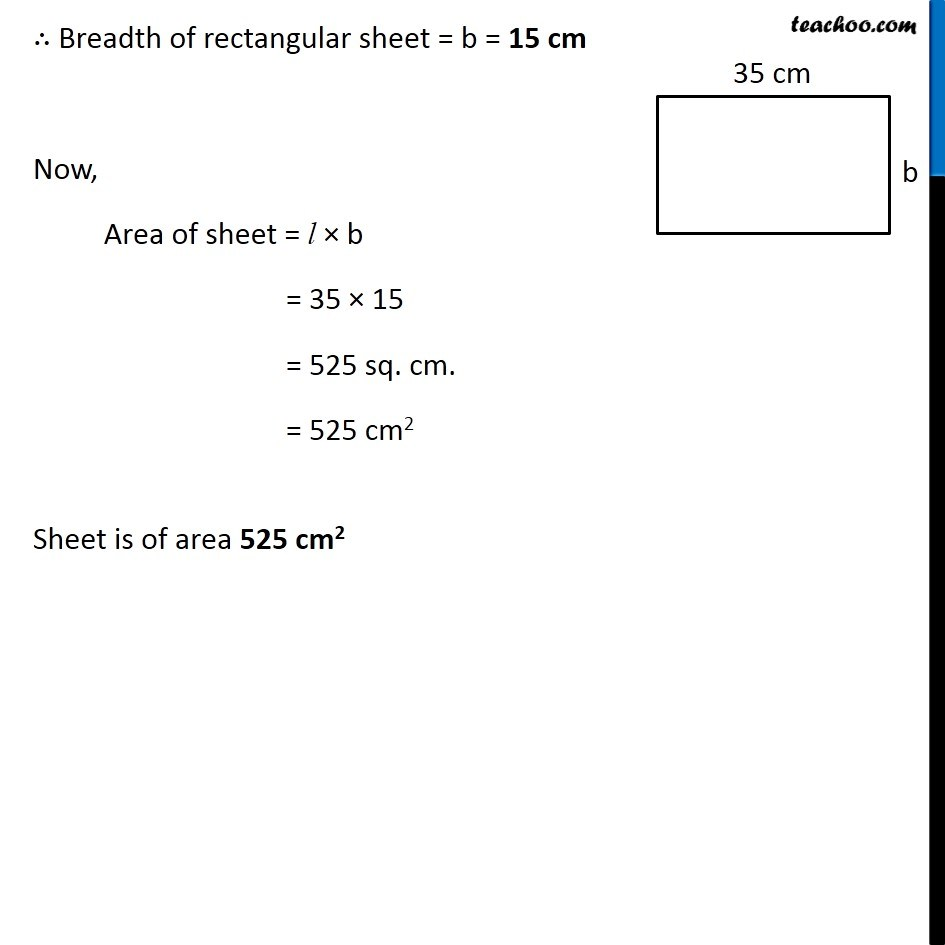Area of Square and Rectangles - Worksheet

Chapter 9 Class 7 Perimeter and Area
Serial order wiseLearn in your speed, with individual attention - Teachoo Maths 1-on-1 Class

### Transcript

Question 4 The perimeter of a rectangular sheet is 100 cm. If the length is 35 cm, find its breadth. Also find the area. Length of rectangular sheet = l = 35 cm Breadth of rectangular sheet = b = ? Given, Perimeter of rectangular sheet = 100 cm 2 (l + b) = 100 2(35 + b) = 100 35 + b = 100/2 b = 50 − 35 b = 15 cm ∴ Breadth of rectangular sheet = b = 15 cm Now, Area of sheet = l × b = 35 × 15 = 525 sq. cm. = 525 cm2 Sheet is of area 525 cm2